# Kelly Criterion For Betting

## How Much Should You Bet In A Game That Favors You ?

Here is an interesting problem.  I’m going to lay out a favorable bet.  You will have the opportunity to place a bet multiple times, and resolve each one before moving onto the next one.  How much should you bet each time so that the you whose luck is exactly average will win the most money ?

Here is the gambling scenario –

• We will flip a fair coin, and if it is heads, you win.
• Before each flip you get to choose how much money you want to bet on that flip
• If you win, you double the amount of money that you bet. If you lose you lose half of the money that you bet.    e. if you bet the full 100 dollars you started with, and you win you have 200 dollars, and if you lose you have 50 dollars.
• You can play this game up to 100 times.

Now clearly this is a favorable bet.  On the first bet, if you bet 100 dollars, you have a 50/50 chance of winning vs losing.  50 percent of 200 dollars plus 50 percent of 50 dollars means the expected value of that bet is 125 dollars, for a gain of 25 dollars.

However just as clearly if you place that bet 100 times you are just as likely to win as many bets as you are to lose those bets.  So if you bet all your money each time, then times that you get lucky and win more times than you lose, for instance win 56 times and lose 44 times, you will win a lot of money.   However the person whose luck is exactly average will win 50 times and double their money, and lose 50 times and cut their money in half, and leave with the same 100 dollars they started with.

We want that average lucky person to win the most money possible, so how much should you bet ?

To simulate that I ran a python program through a couple million people betting different percentages of their bankroll. You can download that python program, the one that generated the charts below, and the associated Excel file here. This chart shows how much money the 50th percentile person would have depending on how much they bet as a percentage of their bankroll.So if you knew that you were going to win exactly 50% of your bets, your optimal bet amount exactly half of your bankroll each time.

Now if you think that you are going to be lucky, and win more than average, or you want to maximize your potential winnings you could bet more.  For a 90th percentile person, you are likely to win 56 bets out of the total 100.  That is a lot better than only winning 50 bets, but it is still a lot of losses.  This is what the winning curve looks like for the 90th percentile personEven though you were in the 90th percentile of luck, betting all your money each time would be a mistake. If you knew that you were going to win exactly 56 of your 100 bets, your optimal bet size would be 68% of your bankroll each time.  So betting more money on a positive expected value game can increase your net expected value, but it would come at an increased risk if you don’t run lucky.

## Even Winnings – In A Game That Favors You

The scenario outlined above may not be the most likely scenario you will encounter.   I have given that scenario some thought because it was in a video game that I played when I was younger.  At the end of each level you were given the opportunity to make a bet 3 times and double or halve the number of coins you had collected in the level each time.  (  Bonus points if you know what Gameboy game I’m referring to)  There are situations in business or with stock option investing that could replicate that, and you could either double your money or lose half your money, but if you are placing bets at a casino the scenario you are most likely to encounter is that you will win or lose the same amount of money.  However your odds of winning and losing may not be 50/50

Let’s say that you had a game where you would win or lose your bet, but that the odds of winning were 55% and you only had a 45% chance of losing.  i.e. imagine that you were betting on red or black on a game of roulette, except that the color distribution favored you instead of the casino. You have the opportunity place this bet 100 times.

Here betting 100% of your money is obviously a bad idea, because when you lose you will lose all of your money, and you will lose almost half the time.  The curve of winnings vs bet rate looks like this for the 50th percentile lucky personAs it turns out, the best amount to bet here is 10% of your bankroll each time.

That 10% number is suspiciously the same as the amount of advantage that you have in the game, i.e. the difference between 55% win rate and 45% loss rate.

If we run the simulation again with a 60% win rate, and a 65% win rate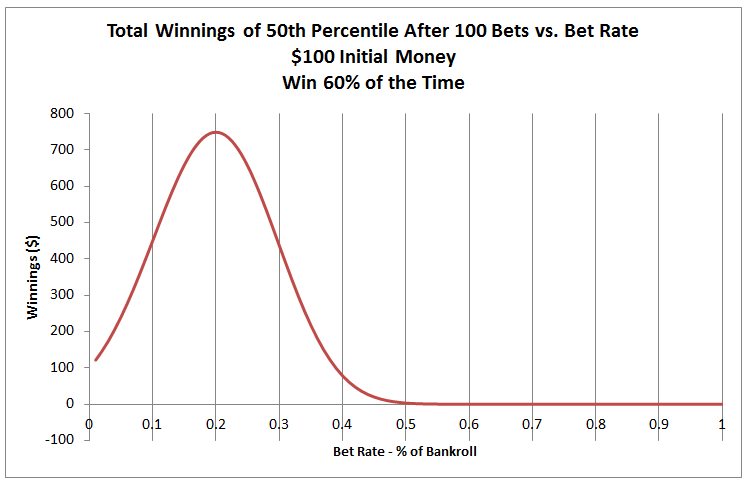We see that the best bet amount for 60% is to bet 20% of your bankroll, and the best bet amount for a 65% win rate is to bet 30% of your bankroll.    It turns out that the best bet is exactly the advantage that you have in the game.

## Kelly Criterion

It turns out that this optimal amount to bet was first discovered in 1956, and is known as the “Kelly Criterion”.   This was named after John Larry Kelly, who was an associate of the famous Claude Shannon, who went on to use this formula as well as other mathematical techniques to make a substantial amount of money in the casinos and in Wall Street.

The Kelley Criterion equation is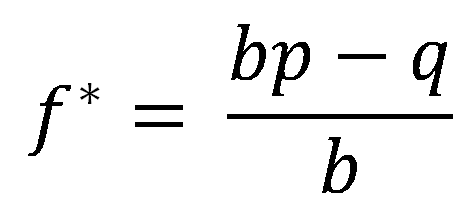Where

• f* is the percentage of the current bankroll to bet
• p is the probability of winning the bet
• q is the probability of losing the bet ( 1 – p )
• b is the net odds received on the bet. e. “b to 1”.  So for a flip of the coin, where you bet 1 and get 2 if you win (your original 1 + 1)  b would be 1.   If you are betting 1 dollar and get 6 if you win, i.e. 5 to 1 odds,  b is 5

This turns out to match our results exactly.  For the first example where we got either double or half, b is 2, and p and q are both .5.  The equation becomes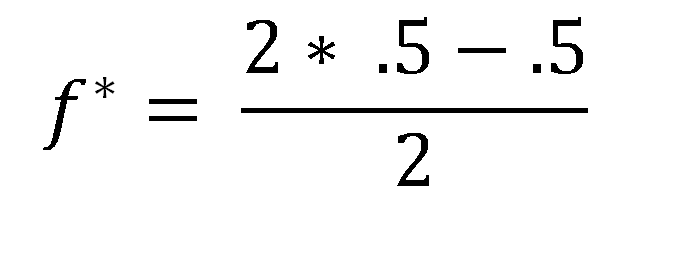And f* is calculated to be .25 = 25% of our bankroll as the optimal bet.   Now the graph indicated that we should be betting 50% of the bankroll.   However since we could only lose half of that 50% of the bankroll in the double or half bet, that is equivalent to betting 25% of the bankroll when using the same terminology as the Kelly Criterion.

For the bets where our payoff is the same as our bet, and our odds of winning are either 55%, 60%, or 65%, b is 1, and an example equation becomes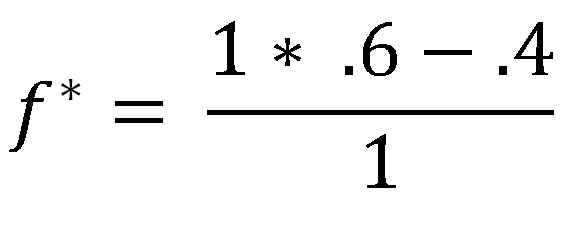Which shows that we should be betting 20% of the bankroll for the 60% chance of winning case, which is exactly the same as the python code determined.

For most real world scenarios, where you are betting against the house which has a house edge, f* becomes negative, which means that you shouldn’t be playing that game.  Truthfully it means that you should take the other side of the wager, become the house, and make them bet against you!

# Gambler’s Ruin

People have come up with many different betting systems over the years. One of these, the Martingale betting system, is interesting because it illustrates how a high level understanding of a concept can be insufficient.

## The Martingale betting system as follows:

• I will start by betting some small amount on a wager that is as close to 50/50 as I can get. So assume I’m betting \$1 on a coin flip.
• Anytime I lose, I’ll double the bet
• Anytime I win, I will start over at \$1
• Since I’m always increasing the stakes, anytime that I win it means that I will have cleared all my loses, and won an additional dollar. For instance, If I lose \$1, \$2, \$4, then win \$8 I will have lost 7 dollars, but then won 8 dollars for a net gain of 1 dollar

What the bettor appears to have is a system that guarantees them small winnings. They will steadily be winning the 1 dollar net bets.

Clearly this is not the case, from Gambler’s Ruin we know that you cannot win any negative expected value game long term. So the Martingale system is not a betting system that you should use. But why is it bad?

There is a surface issue, and an underlying math issue.

## The First Problem With The Martingale – Bet Doubling System

On the surface, one problem is that there isn’t really a game out there that is 50/50 odds you can bet on. Betting red/black in roulette is about as close as you come, but even there your odds of winning are 16/33 or 16/34 depending on the 0’s

## The Main Problem With The Martingale – Bet Doubling System

The underlying math issue is more interesting. It boils down to the fact that you cannot keep doubling your bet forever. At some point you hit either the limit of your bankroll, or the house limit. And in any finite money scenario, all you have done is set up a situation where you frequently win a little bit of money, but infrequently lose a lot of money.

So how does that work ? Let’s give an example with different amounts of bankroll.

Let’s say that you have 7 dollars total. This means that you can keep betting up to the 4 dollar limit, and if you lose the 4 dollar bet you will have lost everything. That gives you a total of 3 bets, at 1, 2, 4, before you lost.   How likely is this to happen ?

This tree illustrates the probabilitiesIf you play this game 8 times, then 7 times you will win 1 dollar, and one time you will lose 7 dollars. The net expected value from this is zero, which is exactly what you would expect since you are assuming a 50/50 game.

If you have 63 dollars, you can run through it and find that you have a 1 in 64 chance of losing the 63 dollars, and a 63 in 64 chance of winning 1 on any give series of bets.

## Your Chance Of Going Broke Is Directly Proportional To Your Bankroll

Assuming 50/50 odds, and that you have a power of 2 bankroll, your chance of going broke is exactly equivalent to your bankroll. Do you have a 1023 dollar bankroll? Then you will go broke 1 in 1024 times. Do you have a 4095 dollar bankroll? You will go broke 1 in 4096 series of bets.

What this strategy does is shift the probability curve, but the total probability remains the same. If you have a 63 dollar bankroll, and you bet 1 dollar 64 times, keeping your bet to 1 dollar regardless of if you won or lost, your probability curve would look like thisWhich can be calculated from the binomial distribution.   Doing that betting strategy, the “Normal” strategy of betting the same amount every time would give you practically zero chance of losing all your money after 64 bets.

If instead you went with the bet doubling strategy, and placed 64 strings of bets this is what the probability curve would look like.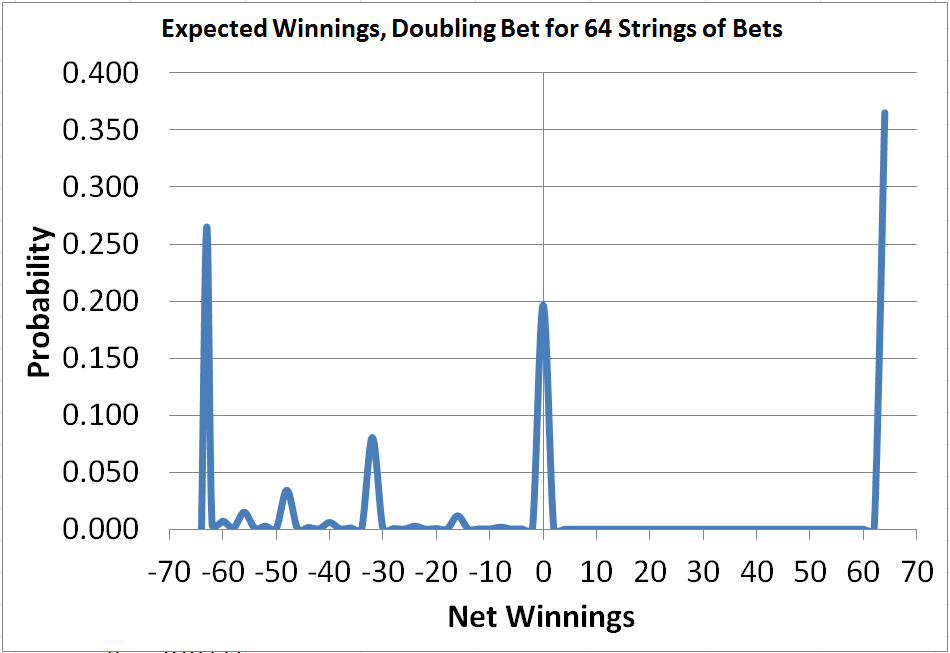I generated these odds plot with a this python program & this Excel file simulating 1 million gambling outings.   Assumptions made were

• You would place 64 strings of bets. With a string ending either when you won your bet, or couldn’t double the bet anymore
• If you lost all your money then you were done
• If you lost and couldn’t double your bet, but you still had some money you would start again at a 1 dollar bet

This chart is much different than the binomial based chart. Most of the probability is pushed to the edges of the chart, so you are most likely to either walk away having won 64 dollars, or having lost your 63 dollar bankroll.   There are other options, such as winning 30 dollars, but then losing your 64 dollar bet and ending up down ~ 34 dollars

The total expected value of that chart, which is the cumulative probability multiplied by net winnings, is zero.

So while Martingale strategy of doubling the bet strategy isn’t a surefire winning technique, it does change the outcome so that instead of being likely to win or lose a little bit, you are most likely to win or lose a lot.